Solution - The NAFEMS Benchmark Challenge 07

February 21st, 2017Often, when faced with a commercial FE system, the engineer will find that it contains a veritable plethora of beam elements each purporting to be suitable for different type of beam. Different beams will typically be based on different beam theories and different FE formulations of the particular theory. For a given beam problem, the different elements will often produce quite different results and often mesh refinement is required in order to home in on the theoretical solution.

This challenge presented two problems, the first of which was used as a software verification problem. Based on the mesh independence of the p-type refinement study conducted on the first problem, the same mesh was used for a design problem in which the load was moved from the center to the three-quarter point. However, the results for the design problem created using the software function for tabulating element data showed moments different to those reported at the nodes. The difference observed in the results led to the challenge of determining the quantities of engineering interest, maximum deflection and moment, for the design problem.

Two Beam Theories

The primary actions seen in beams are shear and bending. For ‘thin’ beams, where the span to thickness ratio is large, the deformations are predominantly due to bending. However, as the span to thickness ratio decreases then deformations due to shear become significant and need to be accounted for both in terms of deflections. The Euler-Bernoulli (EB) theory ignores shear deformation and is thus appropriate to ‘thin’ beams whereas the Timoshenko theory includes a representation of shear deformation and is thus appropriate for thicker beams.

The kinematics of the two beam formulations differ. Both formulations assume that plane sections remain plane but whereas the EB formulation assumes that sections normal to the neutral axis in the undeformed state remain so, in the deformed state, this condition is relaxed for the Timoshenko (T) formulation as illustrated in Figure 2.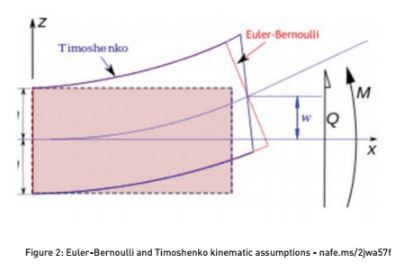Figure 2: Euler-Bernoulli and Timoshenko kinematic assumptions - nafe.ms/2jwa57f

Theoretical Solutions to the Design Problem

The EB solution for the design problem is available in many structural engineering texts and is presented in Figure 3.

Figure 3: Euler-Bernoulli solution to the Design Problem - nafe.ms/2jw1Vvu

The theoretical solutions for the design problem using the two-beam theories are shown in Table 1.  In addition to the significant increase in the displacement under the load with shear deformable theory, the statics, in terms of the shear forces and moments, also change.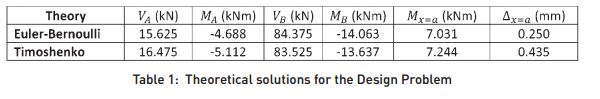The theoretical displaced shapes (exaggerated by scale) for the two theories are shown in Figure 4.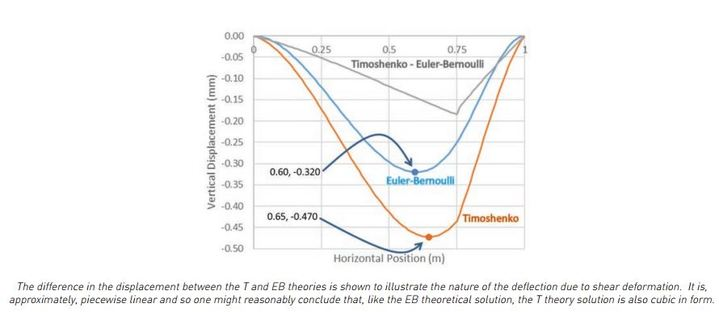Figure 4: Theoretical Displaced shapes for the Design Problem

Finite Element Solutions to the Design Problem

Many finite elements have been formulated based on the two beam theories already discussed. Perhaps the most common beam element based on the EB theory is the two-noded, Hermitian element which interpolates displacement as a cubic polynomial, i.e., it is capable of modelling the linear bending moments of the design problem exactly. In terms of the T theory then there is more variation in the formulation of available elements. The most widely available element is, perhaps, that based on the Mindlin formulation. This element is often of variable degree and interpolates both the displacement and rotations in the same manner, e.g., if the element is a linear element (p=1) then both displacements and rotations are interpolated linearly.  The FE code used to generate the results for this response was of the Mindlin formulation with linear, quadratic and cubic degrees.

It is worth noting that a finite element system should only require a single beam element if the same element could be used reliably for both ‘thick’ and ‘thin’ beams.  There is, however, a numerical issue with conforming (displacement) finite elements (CFE) formulated on T theory when the beam becomes ‘thin’. This issue is known as shear-locking and it can pollute the results of ‘thin’ beams. For this reason, many FE systems offer elements based on both beam theories and this at least allows the engineer to compare the results produced for both theories and confirm whether or not shear-locking is influencing the results.

FE results, using a two element mesh of variable degree Mindlin elements, for both the problems considered in the challenge are presented in Tables 1 and 2.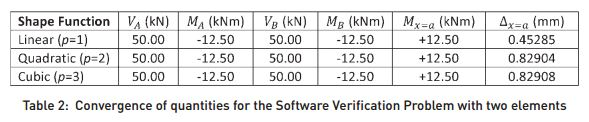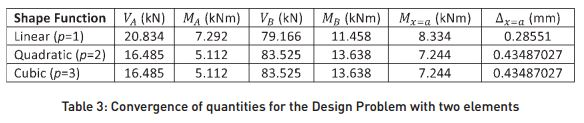It is seen (Table 2) that whilst the shear forces and moments do not change with p-type refinement, the displacements under the load do. There is a significant change between p=1 and p=2 and only a small change, in the fifth significant digit, for p=3. The assumption, made in the challenge, that mesh independence was obtained for p=1, is clearly erroneous.

For the design problem, with the load at the three-quarter position, both shear forces, moments and displacements change as the element degree is increased between linear and quadratic. However, mesh independence does appear to be observed as the results for the cubic element are identical to those for the quadratic element.

Discussion

In the design problem the beam is moderately thick with a span to thickness ratio of 10 and the maximum deflections are 0.32 and 0.47mm respectively for the EB and T theories. Thus, if the EB displacement had been taken, the maximum deflection would have been underestimated by some 30% and this could make the difference between the beam passing and failing an SLS check on maximum deflection.

Theoretical solutions for the design problem were obtained using both beam theories. For both theories, the maximum deflection was seen to occur away from the point load, at 0.60m and 0.65m respectively for the EB and T theories. Many commercial FE systems only report displacements at nodes and unless the maximum displacement occurs at the node then it will not be available to the engineer. The theoretical EB solution for the design problem can, as already noted, be recovered exactly using two cubic Hermitian beam elements. However, in order to recover the maximum displacement the engineer would have had to perform mesh refinement to ‘home in’ on the maximum displacement. This is an example of how poorly implemented post-processing in commercial software can frustrate the engineer’s task.  Had the engineer (erroneously) used an element based on EB theory and taken the maximum displacement from a two-element model then he would have obtained 0.25mm which is almost 50% below the correct value! Of course, if one is adopting an inappropriate mathematical model in addition to not picking up the maximum displacement then simulation governance, the matching of numerical simulation with measured results, will be impossible.

The design problem is hyperstatic (statically indeterminate) thus the different kinematic assumptions of the two beam theories, which result in different beam stiffnesses, also, in addition to the displacements, lead to the different forces and moments. Both sets are, however, in equilibrium with the applied load. The maximum moment for both theories is at the right-hand support and it is seen that the EB theory predicts a moment of 14.06kNm whereas the T theory is about 3% less at 13.64kNm. In an Allowable Stress Design approach, this difference would lead to different factors of safety but in a ULS calculation of the plastic limit load, the collapse load would be unchanged.

For this response, a variable degree Mindlin element was used to model the Timoshenko beam theory. The advice offered by the vendor for this element is that the quadratic (p=2) element is capable of representing linearly varying bending moments exactly. The results almost bear this out except that there is a small difference in the displacement under the load for the Software Verification Problem – see Table 2 – as the degree is increased from quadratic to cubic. The quality of the result for the p=2 Mindlin element is though somewhat surprising since we know, from the theoretical solution shown in Figure 4, that the displacement field is more or less cubic. In investigating this apparent anomaly further, the displacement for a three-noded, quadratic Mindlin element was compared with the exact Timoshenko solution – see Figure 5.Figure 5: Comparison of theoretical displacements with quadratic Mindlin element

The nodal displacements are, more or less, exact but clearly since they are quadratic then between nodes there is significant discrepancy. The moments inside the element are examined to see whether or not they agree with those reported at the nodes - Figure 6.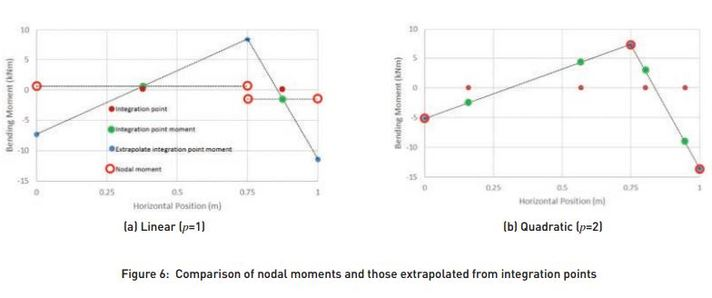In the case of the linear Mindlin element, a single integration point is used and so the variation of the internal moment field is assumed to be constant. This leads to significant differences between the internally generated moments and the nodal moments. Whilst the nodal moments are in equilibrium with the applied load, the internal moments, extrapolated from integration points, are clearly not.  For the quadratic element, two integration points are used and it is seen that these appear to be exact as a linear extrapolation to the nodes leads to the same values as the nodal moments.

Thus, in responding to the challenge, it might be noted that with a two-element mesh of quadratic Mindlin elements, a very close approximation to the theoretically exact solution is obtained.  It is noted, however, that the maximum displacement is not available from this mesh and it has already been noted how this inadequacy might stymy the engineer’s task.  The same is, of course, true for moments. It is rather easy to construct a problem where the maximum moment occurs somewhere between nodes.  Thus both serviceability and ‘strength’ calculations, based on maximum moment, might be compromised for the engineer by the inadequacies of commercial software.

In closing this response, it is important to recognise a potential cause of finite element malpractice when using Mindlin-type elements.  When using a linear element the internal moments (extrapolated from integration points) would not have been the same as the nodal values.  In fact, in this example, they would have been significantly less than the true values - see Figure 6(a).  The erroneously underestimated moments would lead, in an Allowable Stress Design, to an overestimation of the factor of safety and this is clearly of concern to the engineer.

The reason that this point is mentioned is that in some industries the standard technique for assessing structural members is based on internal stress resultants extrapolated from integration points.  As demonstrated in this response, if the degree of the element is not appropriate for the loading seen by the beam then this approach can lead to significantly erroneous stress resultants that would, under code assessment, give an erroneous view of the safety of the structure.

The solution to this potentially significant issue, is to always use stress resultants calculated directly at the nodes from the basic equilibrium equations.  These are guaranteed to be in equilibrium with the applied loads even if the degree of the element is inappropriate to the applied loading, and provided sufficient mesh refinement (either/both p-type and h-type) has been undertaken then these resultants will form an appropriate set on which the structure can safely be assessed.

Download all the Benchmark Challenges and their solutions available so far in the Education and Training Working group section of the NAFEMS website.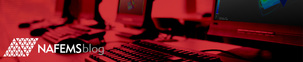Empty List

Sorry nothing to list